Formation and order of matrix

Chapter 3 Class 12 Matrices
Concept wise

Let’s consider the matrix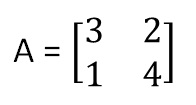It has 2 rows & 2 columns

So, we write the order as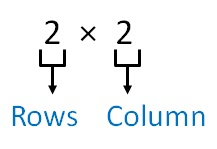And,

3, 2, 1, 4 are elements of matrix A

We write the matrix A as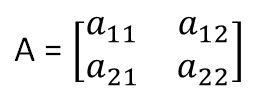Where

a 11 → element in 1st row, 1st column

a 12 → element in 1st row, 2nd column

a 21 → element in 2nd row, 1st column

a 22 → element in 2nd row, 2nd column

So,

a 11 = 3

a 12 = 2

a 21 = 1

a 22 = 4

For matrix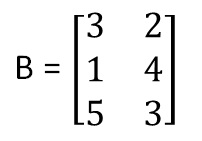It has 3 rows & 2 columns

So, the order is 3 × 2.

We write matrix B as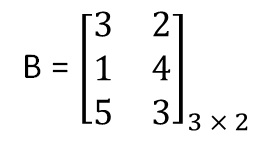Similarly,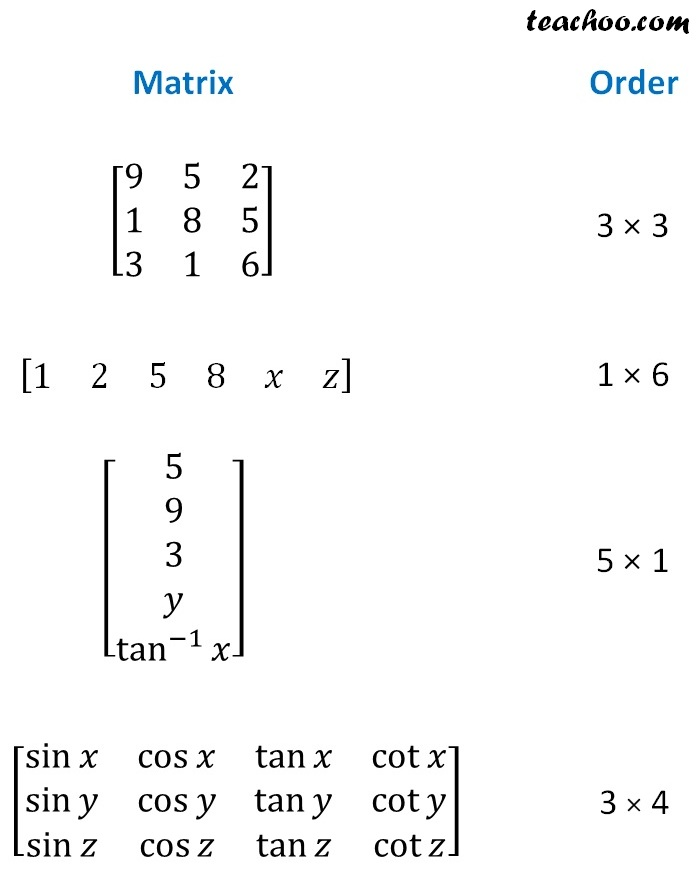## a ij = i + j

A 4 × 3 matrix looks like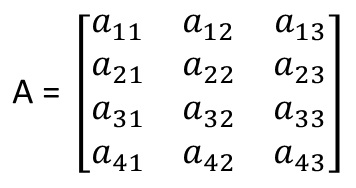Now,

a 11 = 1 + 1 = 2

a 12 = 1 + 2 = 3

a 13 = 1 + 3 = 4

a 21 = 2 + 1 = 3

a 22 = 2 + 2 = 4

a 23 = 2 + 3 = 5

a 32 = 3 + 2 = 5

a 33 = 3 + 3 = 6

a 41 = 4 + 1 = 5

a 42 = 4 + 2 = 5

a 43 = 4 + 3 = 7

So, our matrix is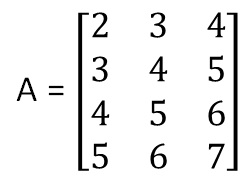Learn in your speed, with individual attention - Teachoo Maths 1-on-1 Class

### Transcript

A = [■8(3&2@1&4)] 2 × 2 Rows Column And, 3, 2, 1, 4 are elements of matrix A A = [■8(𝑎_11&𝑎_12@𝑎_21&𝑎_22 )] B = [■8(3&2@1&4@5&3)] B = [■8(3&2@1&4@5&3)]_(3 × 2) Matrix Order [■8(9&5&2@1&8&5@3&1&6)] 3 × 3 [■8(1&2&5&8&𝑥&𝑧)] 1 × 6 [■8(5@9@3@𝑦@tan^(−1)⁡𝑥 )] 5 × 1 [■8(sin⁡𝑥&cos⁡𝑥&tan⁡𝑥&cot⁡𝑥@sin⁡𝑦&cos⁡𝑦&tan⁡𝑦&cot⁡𝑦@sin⁡𝑧&cos⁡𝑧&tan⁡𝑧&cot⁡𝑧 )] 3 × 4 A = [■8(𝑎_11&𝑎_12&𝑎_13@𝑎_21&𝑎_22&𝑎_23@𝑎_31&𝑎_32&𝑎_33@𝑎_41&𝑎_42&𝑎_43 )] A = [■8(2&3&4@3&4&5@4&5&6@5&6&7)] A = [■8(2&3&4@3&4&5@4&5&6@5&6&7)]